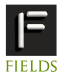11-15 July 2011 INTERNATIONAL CONFERENCE ON SCIENTIFIC COMPUTATION AND DIFFERENTIAL EQUATIONS SciCADE 2011 hosted by the Fields Institute, held at the University of TorontoProgram New Talent Award Toronto Information Contact Us gensci(PUT_AT_SIGN_HERE)fields.utoronto.caMINISYMPOSIA Spatial Error Estimation and Grid Refinement Techniques for the Numerical Solution of PDEs organized by Ronald Haynes, Memorial University of Newfoundland Paul Muir, Saint Mary's University

The Method-Of-Lines is an important approach for the numerical solution of partial differential equations (PDEs). Based on a given spatial grid, the PDE is discretized, leading to a system of time dependent ordinary differential equations (ODEs) or differential-algebraic equations (DAEs). For PDEs in one spatial dimension, standard techniques for discretization, error estimation, and grid refinement for boundary value ODEs are also relevant. This mini-symposium will bring together researchers who will discuss recent work in the method-of-lines approach for the numerical solution of PDEs, with a focus on spatial error estimation and grid refinement techniques

 SPEAKERS Ronald Haynes Memorial University of Newfoundland Domain Decomposition approaches for grid generation via the Equidistribution Principle (slides of talk) Adaptively choosing an underlying grid for computation has proven to be a useful, if not essential, tool for the solution of boundary value problems and partial differential equations. One way of generating adaptive meshes is through the so-called equidistribution principle (EP). In one spatial dimension the required mesh can be obtained through the solution of a nonlinear BVP. In this talk I will review the idea of EP and consider the solution of the resulting BVP via domain decomposition including classical, optimized and optimal Schwarz iterations. Felix Kwok University of Geneva Accuracy and Stability of a Predictor-Corrector Crank-Nicolson Method with Many Subdomains (slides of talk) Many techniques for parallelizing the solution of time-dependent PDEs using domain decomposition ideas have been developed over the past 20 years. One such class of methods uses a predictor-corrector strategy, where variables along subdomain interfaces are first predicted using an explicit method, and the remaining variables are solved implicitly in parallel, using the predicted interface values as boundary conditions. In this talk, we analyze the truncation error and the stability of a predictor-corrector Crank-Nicolson method proposed by Rempe and Chopp (SISC 2006), which formally has second-order accuracy in time for a fixed spatial grid. Paul Muir Saint Mary's University Efficient Interpolant-based Spatial Error Estimation for B-Spline Collocation Solutions of PDEs (slides of talk) BACOL, recently developed collocation software for 1D parabolic PDEs, has been shown to be efficient, reliable and robust, especially for problems with solutions exhibiting sharp layers. It features adaptive control of estimates of the spatial and temporal error. While the BACOL spatial error estimate is generally quite reliable, it involves the expensive computation of two collocation solutions of orders p and p+1. This talk will discuss recent work investigating more efficient spatial error estimation algorithms based on (i) an order p+1 (superconvergent) interpolant and (ii) an order p interpolant whose error agrees asymptotically with the order p collocation solution error. Natesan Srinivasan Indian Institute of Technology Guwahati Second-Order Uniformly Convergent Hybrid Numerical Scheme for Singularly Perturbed Problems of Mixed Parabolic-Elliptic Type Here, we propose a uniformly convergent numerical scheme for a class of singularly perturbed mixed parabolic-elliptic problems exhibiting both boundary and interior layers. The domain under consideration is partitioned into two subdomains. In the first subdomain, the given problem takes the form of parabolic reaction-diffusion type, and in the second subdomain, it takes an elliptic convection-diffusion-reaction type. The numerical scheme consists of the backward-Euler method for the time derivative, and a hybrid scheme for the spatial derivatives. The numerical method is analyzed on a layer resolving piecewise-uniform Shishkin mesh, and parameter-uniform error estimate is obtained. Numerical examples are carried out to validate the theoretical results.